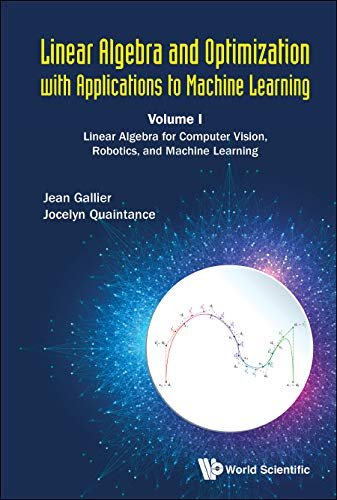# Linear Algebra and Optimization with Applications to Machine Learning: Volume I: Linear Algebra for Computer Vision, Robotics, and Machine Learning

• Length: 824 pages
• Edition: 1
• Publisher:
• Publication Date: 2020-01-15
• ISBN-10: 9811206392
• ISBN-13: 9789811206399
Description

This book provides the mathematical fundamentals of linear algebra to practicers in computer vision, machine learning, robotics, applied mathematics, and electrical engineering. By only assuming a knowledge of calculus, the authors develop, in a rigorous yet down to earth manner, the mathematical theory behind concepts such as: vectors spaces, bases, linear maps, duality, Hermitian spaces, the spectral theorems, SVD, and the primary decomposition theorem. At all times, pertinent real-world applications are provided. This book includes the mathematical explanations for the tools used which we believe that is adequate for computer scientists, engineers and mathematicians who really want to do serious research and make significant contributions in their respective fields.